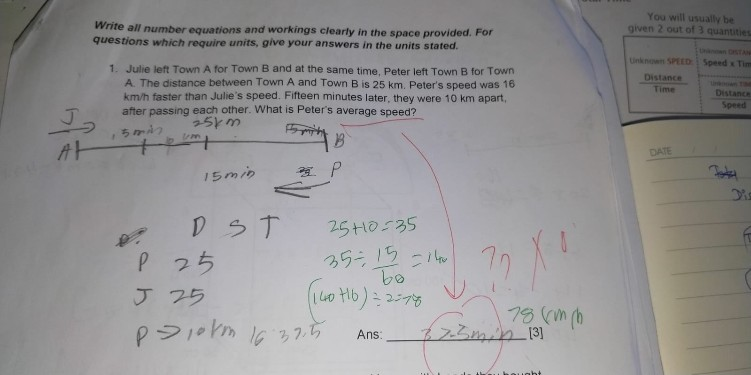# Questioncan you help solution for this problem

1 Answer

# Answer

Total distance covered by both Julie and Peter = 25 + 10 = 35km

Total average speed of both Julie and Peter = 35 / 0.25 = 140 km/h

Given Peter’s speed – Julie’s speed = 16 km/h

Julie’s speed = (140 -16) / 2 = 62 km/h,  Peter’s speed = 62 + 16 = 78 km/h

0 Replies 0 Likes